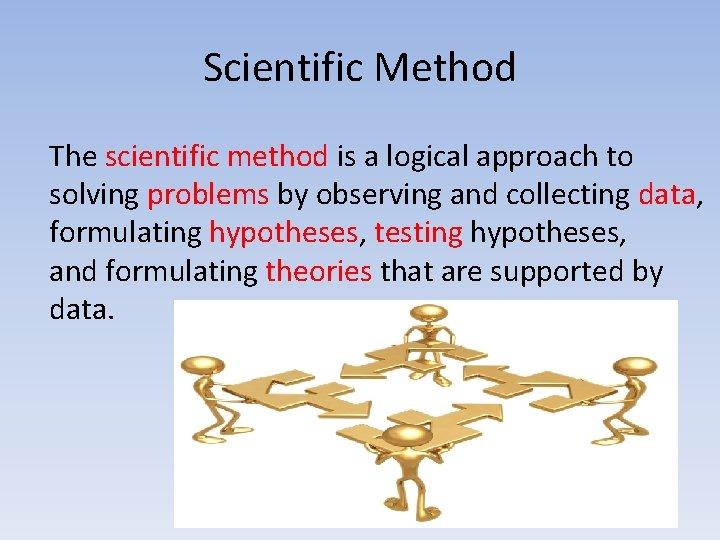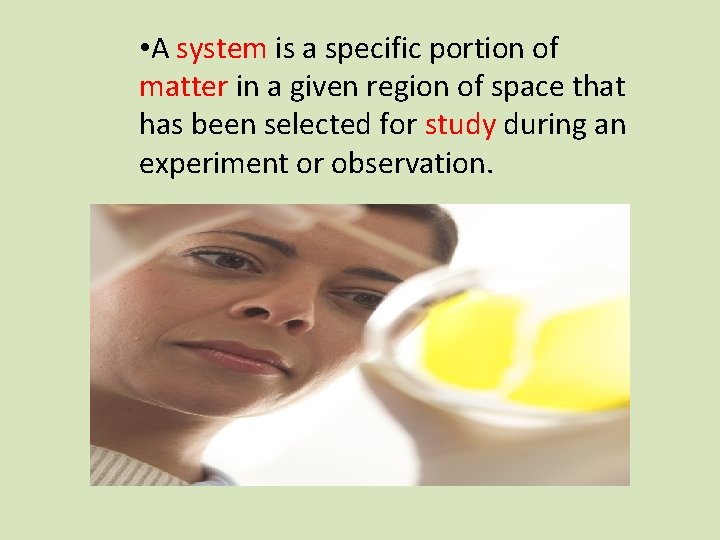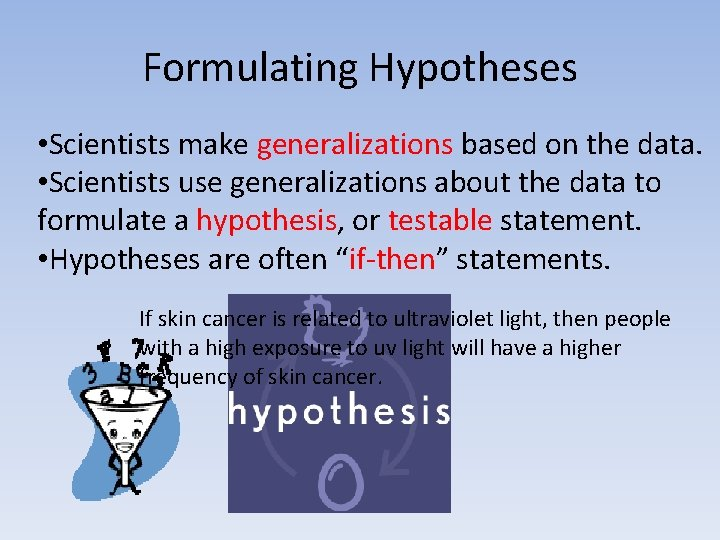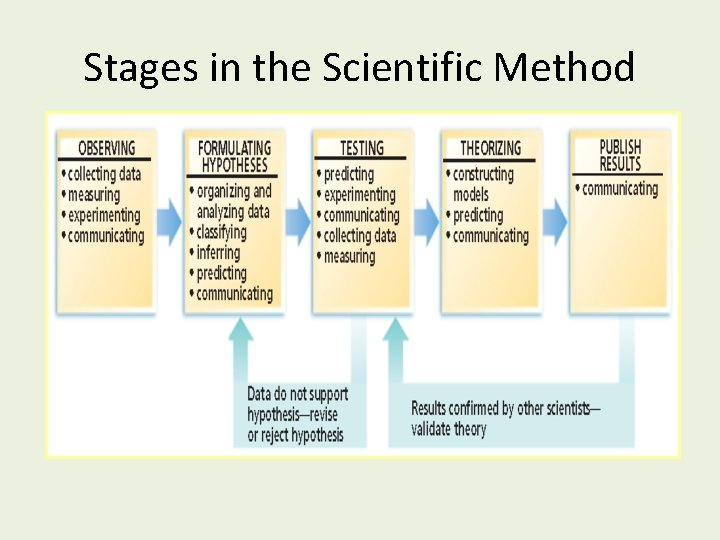# Scientific Method Scientific Method The scientific method is

• Slides: 9Scientific MethodScientific Method The scientific method is a logical approach to solving problems by observing and collecting data, formulating hypotheses, testing hypotheses, and formulating theories that are supported by data.Observing and Collecting Data Observing is the use of the senses to obtain information. • data may be • qualitative (descriptive) • quantitative (numerical)• A system is a specific portion of matter in a given region of space that has been selected for study during an experiment or observation.Formulating Hypotheses • Scientists make generalizations based on the data. • Scientists use generalizations about the data to formulate a hypothesis, or testable statement. • Hypotheses are often “if-then” statements. If skin cancer is related to ultraviolet light, then people with a high exposure to uv light will have a higher frequency of skin cancer.Testing Hypotheses • Testing a hypothesis requires experimentation that provides data to support or refute a hypothesis or theory. • Controls are the experimental conditions that remain constant. • Variables are any experimental conditions that change.Theorizing A model in science is more than a physical object; it is often an explanation of how phenomena occur and how data or events are related. • visual, verbal, or mathematical • example: atomic model of matterA theory is a broad generalization that explains a body of facts or phenomena. • example: atomic theoryStages in the Scientific Method